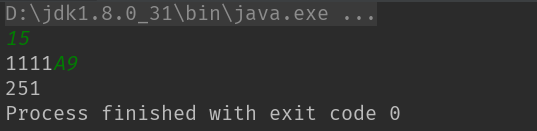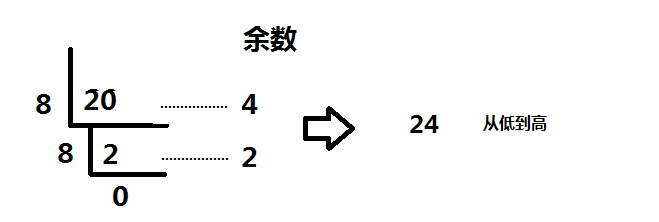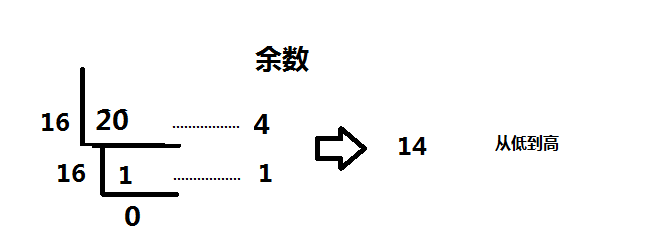• java进制转换（十进制转八进制，十进制转二进制，十六进制转八进制） 这几天在复习C语言的数据结构栈和队列那一章的时候，看到利用栈的特性FILO实现的进制转换十分简洁 想起了java中实现栈的操作十分方便（不用...
java进制转换（十进制转八进制，十进制转二进制，十六进制转八进制）

这几天在复习C语言的数据结构栈和队列那一章的时候，看到利用栈的特性FILO实现的进制转换十分简洁

想起了java中实现栈的操作十分方便（不用自己写.h文件，内部util.Stack包已经封装好）

所以用这个来写一个进制转换作为记录

十进制怎么转化为二进制呢？

    public void Dex2Bin(int n){
int x;
Stack<Integer> stack = new Stack<>();
while(n>0){
x=n%2;
stack.push(x);
n/=2;
}
while(!stack.empty()){
int num = stack.pop();
System.out.format("%d",num);
}
}

首先是获取被转换的数据n，创建一个栈，new即可，自动初始化（对应C语言中的top指针指向base）

当n>0的时候，不断的：

除以2取余 x=n%2，取余的数字压栈
数字除以2取整 n/=2
这样就完成了对一个数字进制转换的过程。

输出的时候只要对stack不断的pop即可

同理十进制转八进制也是如此

    public void Dex2Oct(int n){
int x;
Stack<Integer> stack = new Stack<>();
while(n>0){
x=n%8;
stack.push(x);
n/=8;
}
while(!stack.empty()){
int num = stack.pop();
System.out.format("%d",num);
}
}

和二进制转换类似，不多赘述

十六进制怎么转化为八进制呢？

    public void Hex2Oct(String n) {
int hex2dex = 0;
int reIndex = 0;
/*十六进制转十进制*/
for (int i = n.length()-1; i >= 0; i--) {
if(Character.isDigit(n.charAt(i))&&
Integer.parseInt(String.valueOf(n.charAt(i))) >= 0 &&
Integer.parseInt(String.valueOf(n.charAt(i))) <= 9){
hex2dex += Integer.parseInt(String.valueOf(n.charAt(i))) * Math.pow(16, reIndex);
} else {
switch (n.charAt(i)) {
case 'A':
hex2dex += 10 * Math.pow(16, reIndex);
break;
case 'B':
hex2dex += 11 * Math.pow(16, reIndex);
break;
case 'C':
hex2dex += 12 * Math.pow(16, reIndex);
break;
case 'D':
hex2dex += 13 * Math.pow(16, reIndex);
break;
case 'E':
hex2dex += 14 * Math.pow(16, reIndex);
break;
case 'F':
hex2dex += 15 * Math.pow(16, reIndex);
break;
}
}
reIndex++;
}
//十进制转八进制
Dex2Oct(hex2dex);

}

这里说实话，我个人觉得写得很啰嗦，希望能提出改进意见，主要是在char转换位int的时候有些麻烦

上面的算法分为两个部分：1、十六进制字符串转换为对应的十进制，完成了从字符串到数字的转换

2、十进制转换为八进制

首先是逐个解析十六进制字符串，采用倒序循环的方式。

同时设置一个从0开始递增的辅助变量reIndex，帮助算法完成对最后一个字符的乘以16的N次方的行为。

解析的时候，首先判断这个字符串是不是一个数字型比如‘9’：

若是，对这个字符转换为数字9之后，乘以相应的16的N次方；
若不是，对这个数字单独辨识，必定是ABCDEF其中一个，在对这些数字表示的十进制数值进行运算操作。
得到十六进制转换的十进制数字之后，对这个数字进行上面已经完成的十进制转八进制的运算，只要传入数字即可获得答案。

总结

算法特点，利用栈的特点解决进制转换很方便
转换出来的二进制没有前缀00...

贴完整代码：

样例输入：先输入十进制转换二进制的十进制数字：比如15

转换完成之后输入一个16进制字符，转换为8进制import java.util.Scanner;
import java.util.Stack;
/**
* Page Description:
* User: Yelihu
* Date: 2018-12-19
* Time: 上午 9:16
* Function:
*/
public class Main {
public void Dex2Bin(int n){
int x;
Stack<Integer> stack = new Stack<>();
while(n>0){
x=n%2;
stack.push(x);
n/=2;
}
while(!stack.empty()){
int num = stack.pop();
System.out.format("%d",num);
}
}
public void Dex2Oct(int n){
int x;
Stack<Integer> stack = new Stack<>();
while(n>0){
x=n%8;
stack.push(x);
n/=8;
}
while(!stack.empty()){
int num = stack.pop();
System.out.format("%d",num);
}
}
public void Hex2Oct(String n) {
int hex2dex = 0;
int reIndex = 0;
/*十六进制转十进制*/
for (int i = n.length()-1; i >= 0; i--) {
if(Character.isDigit(n.charAt(i))&&
Integer.parseInt(String.valueOf(n.charAt(i))) >= 0 &&
Integer.parseInt(String.valueOf(n.charAt(i))) <= 9){
hex2dex += Integer.parseInt(String.valueOf(n.charAt(i))) * Math.pow(16, reIndex);
} else {
switch (n.charAt(i)) {
case 'A':
hex2dex += 10 * Math.pow(16, reIndex);
break;
case 'B':
hex2dex += 11 * Math.pow(16, reIndex);
break;
case 'C':
hex2dex += 12 * Math.pow(16, reIndex);
break;
case 'D':
hex2dex += 13 * Math.pow(16, reIndex);
break;
case 'E':
hex2dex += 14 * Math.pow(16, reIndex);
break;
case 'F':
hex2dex += 15 * Math.pow(16, reIndex);
break;
}
}
reIndex++;
}
//十进制转八进制
Dex2Oct(hex2dex);

}
public static void main(String[] args) {
Main main = new Main();
Scanner sc = new Scanner(System.in);
int n = sc.nextInt();
//十进制转二进制
main.Dex2Bin(n);
Scanner sc1 = new Scanner(System.in);
String str1 = sc1.nextLine();
//十六进制转八进制
main.Hex2Oct(str1);
}
}


展开全文• 以下程序的输出结果是 main(){ int a=20; printf("%d,%o,%x\n",a,a,a);...1.题目给出了 a=20这个十进制现在我们需要用十进制转八进制，这里我们采用的就是直接除8取余的方法 2.同理对于10进制转16进制我们也是...
以下程序的输出结果是
main(){

int a=20;

printf("%d,%o,%x\n",a,a,a);

}
看到这个题目首先我们要明白%o 和 %x代表的是什么意思

%o代表的是输出该数字的八进制

%x代表的是输出该数字的十六进制

1.题目给出了 a=20这个十进制现在我们需要用十进制转八进制，这里我们采用的就是直接除8取余的方法2.同理对于10进制转16进制我们也是采取除16取余的方法3.相反，如果我们有一个十六进制那么怎么转成十进制、八进制呢？请看下面解释

（1）十六进制转十进制

16进制数的第0位的权值为16的0次方，第1位的权值为16的1次方，第2位的权值为16的2次方……

例：2AF5换算成10进制:

用竖式计算：

第0位： 5 * 16^0 = 5

第1位： F * 16^1 = 240

第2位： A * 16^2= 2560

第3位： 2 * 16^3 = 8192

直接计算就是：

5 * 16^0 + F * 16^1 + A * 16^2 + 2 * 16^3 = 10997

（2）十六进制转二进制

由于在二进制的表示方法中，每四位所表示的数的最大值对应16进制的15，即16进制每一位上最大值，所以，我们可以得出简便的转换方法，将16进制上每一位分别对应二进制上四位进行转换，即得所求：

例：2AF5换算成2进制:

第0位： （5）16 = （0101) 2

第1位： （F）16 = (1111) 2

第2位： (A) 16 = (1010) 2

第3位： (2) 16 = (0010) 2

得：(2AF5)16=(0010.1010.1111.0101)2

（3）十六进制转八进制

先将十六进制转为二进制，再将二进制转为八进制


展开全文• 十进制转八进制 例子： 输入： 10 输出： 12 思路：对8进行取模运算并存入栈，直至该数字为0，将栈输出 public static void Dex2Oct(int num){ if (num < 8){ System.out.println("转换后的八进制数为:"+ num);...
十进制转八进制
例子：
输入： 10
输出： 12
思路：对8进行取模运算并存入栈，直至该数字为0，将栈输出
public static void Dex2Oct(int num){
if (num < 8){
System.out.println("转换后的八进制数为:"+ num);
}
int i;
Stack<Integer> stack = new Stack<>();
while (num > 0){
i = num % 8;
stack.push(i);
num /= 8;
}

while (stack.size() > 0){
Integer pop = stack.pop();
System.out.format("%d",pop);
}
}


十六进制转八进制 先将十六进制转为十进制，再将十进制转为八进制
方法：从末尾遍历字符串，权值为0，倒数第二位权值为16，第三位为16*16，依次。。。
使用Math.pow(16,index)，index代表权值
public static void Hex2Oct(String n){
//位数
int reIndex = 0, dexVal = 0;
for (int i = n.length() - 1; i >= 0; i--) {
if (Character.isDigit(n.charAt(i)) &&
Integer.parseInt(String.valueOf(n.charAt(i))) >=0 &&
Integer.parseInt(String.valueOf(n.charAt(i))) <=9){
dexVal += Integer.parseInt(String.valueOf(n.charAt(i))) * Math.pow(16,reIndex);
}else{
switch (n.charAt(i)){
case 'A':
//权值*该位上的数字值
dexVal += 10 * Math.pow(16,reIndex);
break;
case 'B':
dexVal += 11 * Math.pow(16,reIndex);
break;
case 'C':
dexVal += 12 * Math.pow(16,reIndex);
break;
case 'D':
dexVal += 13 * Math.pow(16,reIndex);
break;
case 'E':
dexVal += 14 * Math.pow(16,reIndex);
break;
case 'F':
dexVal += 15 * Math.pow(16,reIndex);
break;
}
}
reIndex ++;
}
//调用上述十进制转八进制
Dex2Oct(dexVal);
}



展开全文leetcode
• 此篇博客是摘抄总结的，巩固基础。...二进制转10进制： int BinarytoDecimal(long long n) { int decimalnumber = 0, i = 0, remainder; while(n!=0) { remainder = n%10; n/=10; decimalnumber +...
此篇博客是摘抄总结的，巩固基础。
上代码：
- 二进制转十进制：
int BinarytoDecimal(long long n)
{
int decimalnumber = 0, i = 0, remainder;
while(n!=0)
{
remainder = n%10;
n/=10;
decimalnumber += remainder*pow(2,i);
i++;
}
return decimalnumber;
}

由于二进制的长度较长，所以定义的是longlong类型。且编写的函数中用到了pow()函数，所以在使用之前需要调用<math.h>头文件。每一步的代码的具体含义可以自行揣度，并不难。
同理，八进制转十进制也可以根据上述代码加以修改便能使用，原理相同。
- 八进制转十进制：
上代码：
int OctaltoDecimal(long long n)
{
int decimalnumber = 0, i = 0, remainder;
while(n!=0)
{
remainder = n%10;
n/=10;
decimalnumber += remainder*pow(8,i);
i++;
}
return decimalnumber;
}

- 十六进制转十进制：
当然十六进制转十进制稍微有点难度，其难点在于输入十六进制时输入的是字符串而不是整数，所以我们必须从字符串着手。
上代码：
int HexadecimaltoDecimal(char a[], int length)
{
int i, j;
int decimalnumber = 0;
for(i=length-1,j=0;i>=0;i--,j++)
{
if(isalpha(a[i]))
decimalnumber += (a[i]-'A'+10)*pow(16,j);
else
decimalnumber += (a[i]-'0')*pow(16,j);
}
return decimalnumber;
}

机制与二进制转十进制的进制类似，但是需要对每个字符进行处理，使每一位都变成意义想通的整数。需要用到<math.h>,<string.h>两个头文件。
- 十进制转二进制
上代码：
int DecimaltoBinary(int n)
{
long binarynumber = 0;int i = 1, remainder;
while(n!=0)
{
remainder = n%2;
n /= 2;
binarynumber += remainder*i;
i *= 10;
}
return binarynumber;
}

其实十进制转二进制还有一种思路，那就是通过定义一个整数数组，把十进制数模2的余数存进去，最后倒叙输出即可。
上代码：
int a;
int DecimaltoBinary(int n)
{

int i = 0, remainder;
while(n!=0)
{
remainder = n%2;
n /= 2;
a[i++] = remainder;
}
for(i=i-1;i>=0;i--)
printf("%d", a[i]);
}

此处需要定义一个全局数组。
- 十进制转八进制
上代码：
int DecimaltoOctal(int n)
{
int octalnumber = 0, i = 1;
while (n != 0)
{
octalnumber += (n % 8) * i;
n /= 8;
i *= 10;
}
return octalnumber;
}

- 十进制转十六进制
这个就稍显麻烦了，网上看到过一种思路，就是将十进制数对16取模，然后用一个数组将每个余数存起来，定义一个函数将数组的每个数转换成十六进制的数码即可
上代码：
#include <stdio.h>

char getnum(int a)
{
switch(a) //进制转换
{
case 0:
return '0';
break;
case 1:
return '1';
break;
case 2:
return '2';
break;
case 3:
return '3';
break;
case 4:
return '4';
break;
case 5:
return '5';
break;
case 6:
return '6';
break;
case 7:
return '7';
break;
case 8:
return '8';
break;
case 9:
return '9';
break;
case 10:
return 'A';
break;
case 11:
return 'B';
break;
case 12:
return 'C';
break;
case 13:
return 'D';
break;
case 14:
return 'E';
break;
case 15:
return 'F';
break;

}
}

int main()
{
int a;
int n,i=0;
scanf("%d",&n);

if(n==0)
{
printf("0");
}

else
{
while(n)
{
a[i++]=n%16;
n/=16;
}

for(i=i-1; i>=0; i--)
{
putchar(getnum(a[i]));
}
}
return 0;
}


至于还有其他的转换，就可以通过上述转换为中间媒介进行转换。


展开全文• 十进制转R进制 十进制转二进制 十进制整数转二进制 十进制整数转换成二进制采用“除2倒取余”，十进制小数转换成二进制小数采用“乘2取整”。 例题： 135D = __ B 解析：如下图所示，将135除以2，得余数，直到...计算机基础
• 数制转换问题，十进制转八进制，十进制转N进制问题数制转换问题
•数据结构 C++
• ----------- android培训、java培训、java...23十进制  0-9逢10进1  023 八进制 0-7 逢8进1 用0开头表示 0x4a2c 十六进制 0-9 A-F 逢16进1 用0x开头表示 二进制 逢2进1 10101010 二进制基本单位是位【字节是由8
• 十进制转换八进制、十六进制输出 #include <stdio.h> int main () { int n; printf("请输入一个十进制数：\n"); scanf("%d", &n); printf("它的八进制是：%o\n",n); printf("它的十六进制是：%x\n", ...
• 十进制转八进制、十六进制 问题描述 　十六进制数是在程序设计时经常要使用到的一种整数的表示方式。它有0,1,2,3,4,5,6,7,8,9,A,B,C,D,E,F共16个符号，分别表示十进制数的0至15。十六进制的计数方法是满16进1，所以...
• import java.util.HashMap; import java.util.Map; /** * Main_17_Decimal_Conversion ... * 十进制转为八进制 */ public class Main_17_Decimal_Conversion { public static void main(String[] args...
• //十进制转八进制 #include"stdio.h" #include"stdlib.h" #define maxsize 100 typedef struct { int data[maxsize]; int top; }link; void initializa(link *s)//初始化函数 { s->top=0; } int empty(link...c语言
• 使用Python内置函数：bin()、oct()、int()、hex()可实现进制转换。先看Python官方文档中对这几个内置函数的描述：bin(x)Convert an integer number to a binary string. The result is a valid Python expression. ......